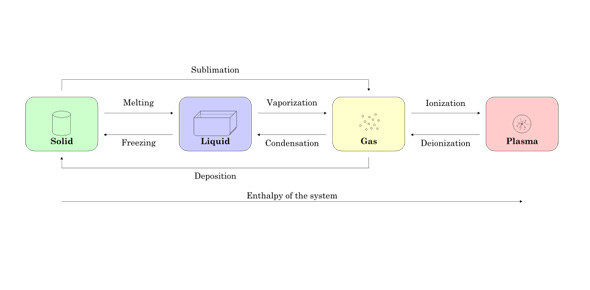# States Of Matter- Graf

14 Questions | Total Attempts: 48SettingsStudents demonstrate their understanding of properties and states of matter. Students show how energy, temperature, volume and pressure are related.

Related Topics
• 1.
How does heat affect the motion of molecules?
• A.

Heat causes molecules to speed up

• B.

Heat causes molecules to slow down

• C.

Heat has no impact on molecular motion

• 2.
As temperature increases, the space between the molecules will...
• A.

Decrease

• B.

Increase

• C.

Stay the same

• 3.
What happens when water changes from a liquid to a vapor in a closed container? Check all the true statements.
• A.

The number of molecules increases.

• B.

The motion of the molecules increases.

• C.

The amount of space between the molecules decreases.

• D.

The motion of the molecules decreases.

• E.

The amount of space between the molecules increases.

• F.

The number of molecules decreases.

• 4.
Why is it possible for large amounts of gases to be stored into small tanks?
• A.

The atoms and molecules of gases are smaller than the atoms and molecules of solids and liquids

• B.

The atoms and molecules in gases do not take up any space

• C.

Gases can be compressed because there is a lot of space between the atoms and molecules

• 5.
In the image below, molecules are moving fast and colliding into one another and into all sides of the container. What state of matter is being represented?
• A.

Solid

• B.

Liquid

• C.

Gas

• 6.
In the image below, molecules are loosely sliding past one another. What state of matter is represented?
• A.

Solid

• B.

Liquid

• C.

Gas

• 7.
In the image below, molecules are vibrating in one spot back and forth. What state of matter is represented?
• A.

Solid

• B.

Liquid

• C.

Gas

• 8.
What happens to molecules in a gas when it cools?  Mark all the right answers
• A.

Molecules contract

• B.

Molecules slow down

• C.

Molecules expand

• D.

The pressure it puts on its container increases

• E.

The pressure it puts on its container decreases

• F.

The molecules gain energy

• 9.
What happens when you put ice into a glass of soda at room temperature? Check all correct answers
• A.

The ice molecules gain energy and speed up

• B.

The ice molecules loose energy and slow down

• C.

The soda molecules gain energy and slow down

• D.

The soda molecules loose energy from bumping into the slow moving ice molecules

• 10.
The phase change that occurs when a gas cools and changes into a liquid is known as...
• A.

Evaporation

• B.

Condensation

• C.

Melting

• D.

Freezing

• 11.
The phase change that occurs when a liquid cools and changes into a solid is known as...
• A.

Evaporation

• B.

Condensation

• C.

Melting

• D.

Freezing

• 12.
The phase change that occurs when a liquid is heated and changes into a gas is known as...
• A.

Evaporation

• B.

Condensation

• C.

Melting

• D.

Freezing

• 13.
The phase change that occurs when a solid is heated and changes into a liquid is known as...
• A.

Evaporation

• B.

Condensation

• C.

Melting

• D.

Freezing

• 14.
Why is it possible to observe all three phases of matter in one setting?
• A.

Because the temperature might be hotter or colder in that setting

• B.

Because different substances have different melting and boiling temperatures

• C.

It would not be possible if the temperature in that setting were the same